NCERT Exemplar - MCQs

Chapter 6 Class 12 Application of Derivatives
Serial order wise

## (C) − 6/7          (D) −6

This question is exactly same Misc 20 MCQ  - Chapter 6 Class 12 - Application of Derivatives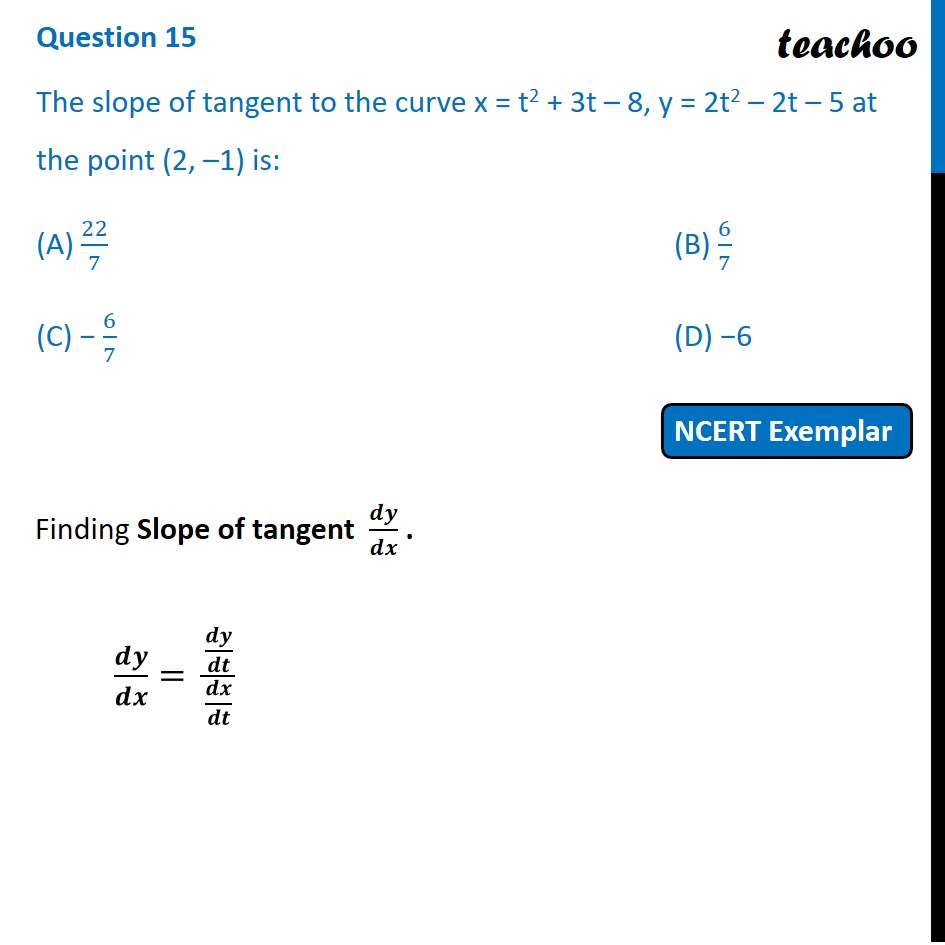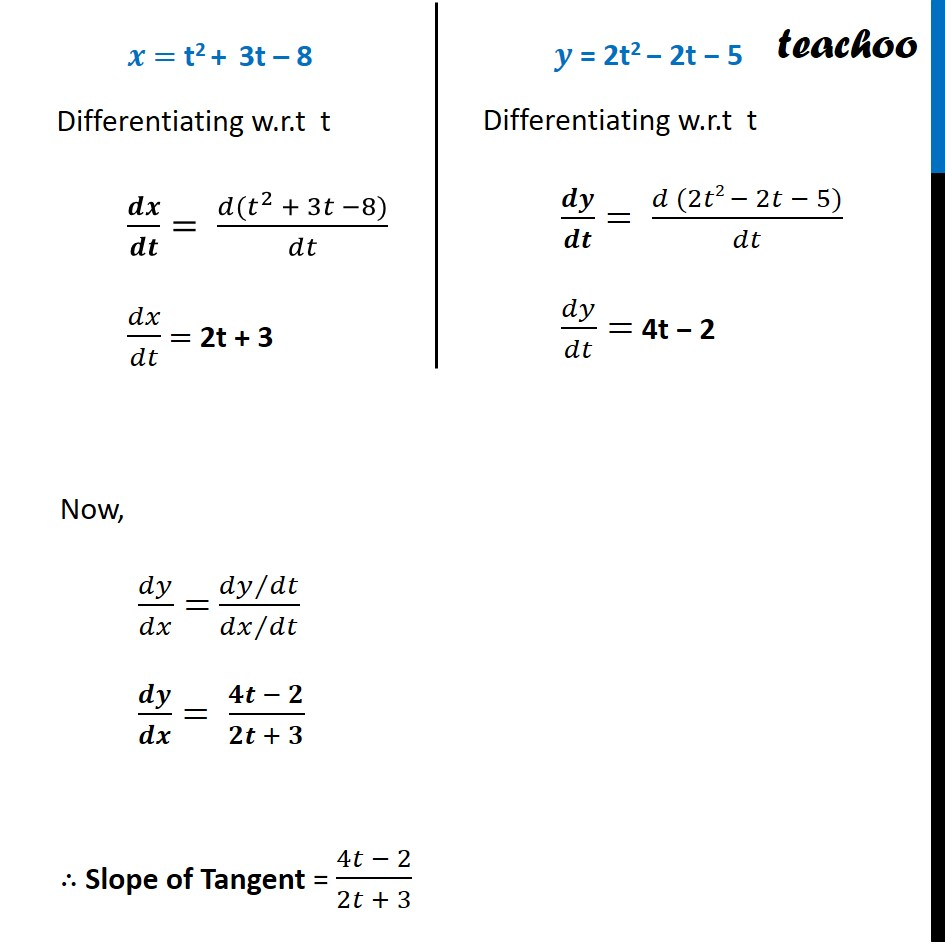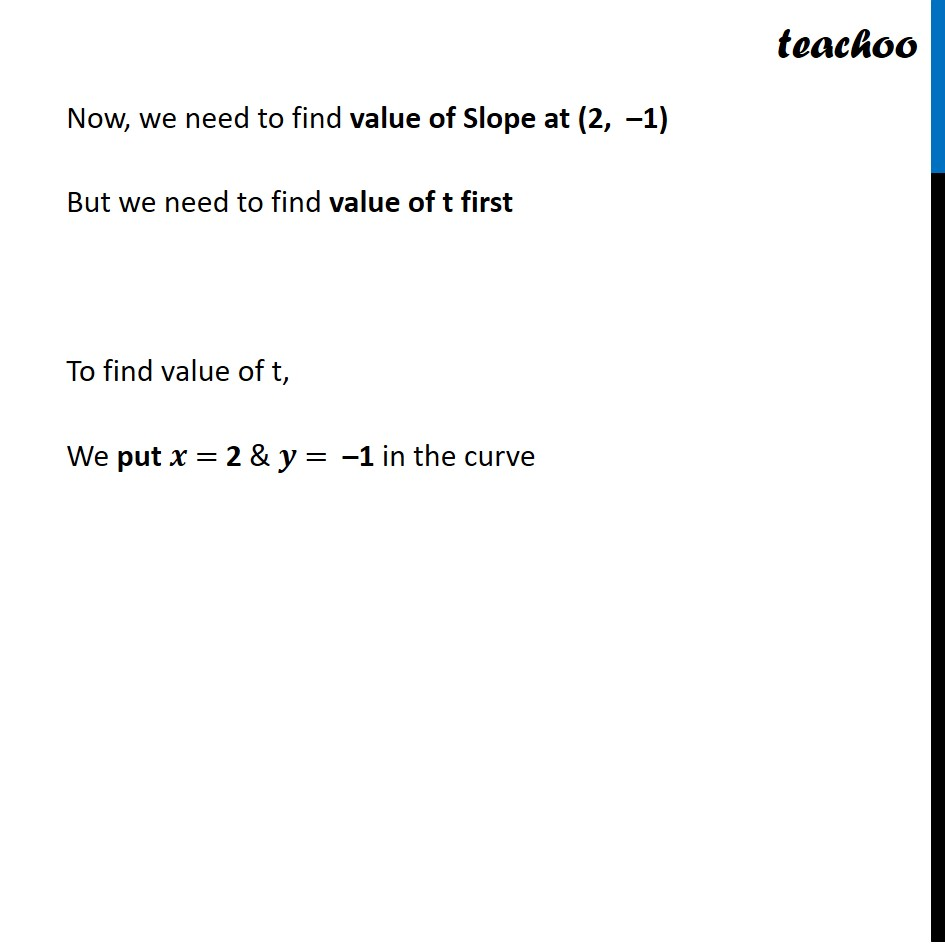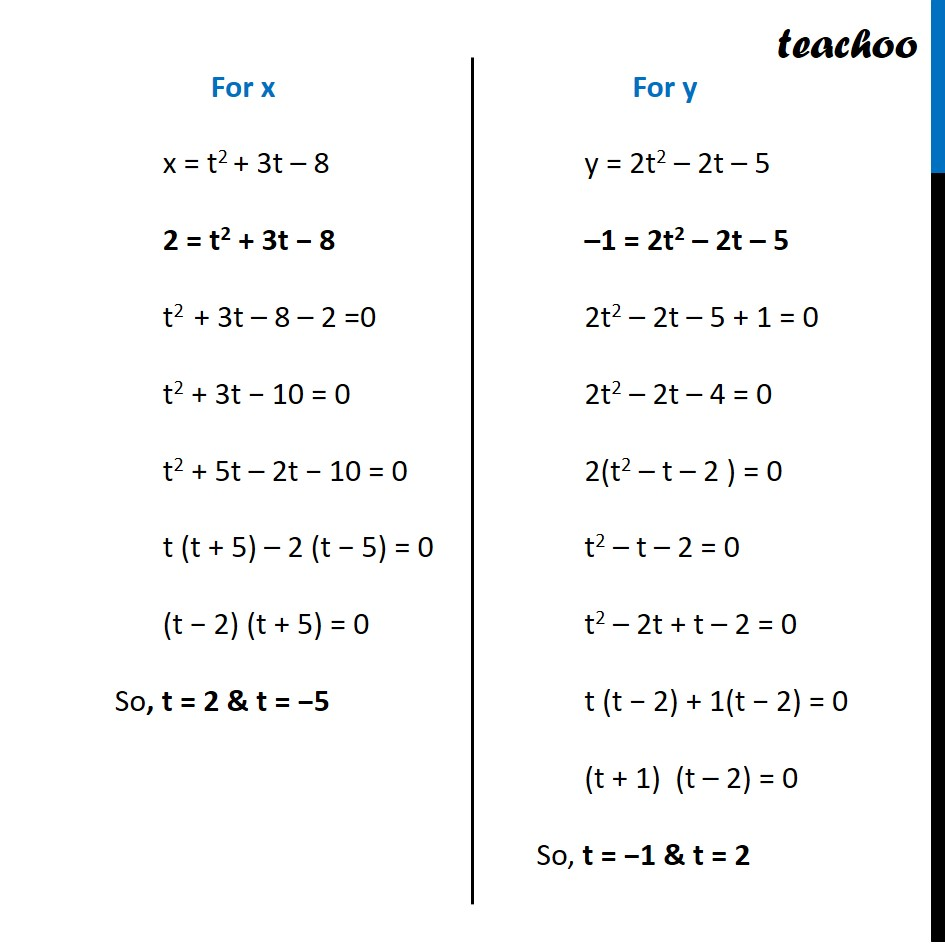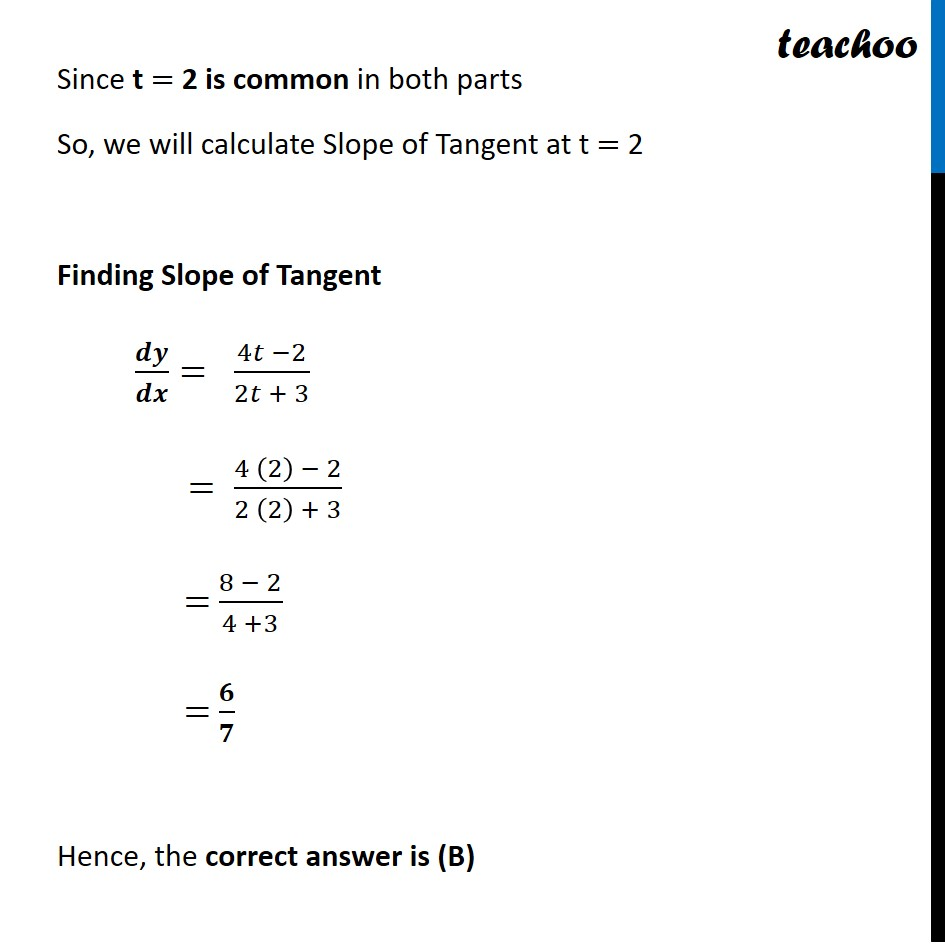Get live Maths 1-on-1 Classs - Class 6 to 12

### Transcript

Question 15 The slope of tangent to the curve x = t2 + 3t – 8, y = 2t2 – 2t – 5 at the point (2, –1) is: (A) 22/7 (B) 6/7 (C) − 6/7 (D) −6 Finding Slope of tangent 𝒅𝒚/𝒅𝒙 . 𝒅𝒚/𝒅𝒙= (𝒅𝒚/𝒅𝒕)/(𝒅𝒙/𝒅𝒕) 𝒙 = t2 + 3t – 8 Differentiating w.r.t t 𝒅𝒙/𝒅𝒕= (𝑑(𝑡^2 + 3𝑡 −8))/𝑑𝑡 𝑑𝑥/𝑑𝑡 = 2t + 3 𝒚 = 2t2 − 2t − 5 Differentiating w.r.t t 𝒅𝒚/𝒅𝒕= (𝑑 (2𝑡2 − 2𝑡 − 5))/𝑑𝑡 𝑑𝑦/𝑑𝑡= 4t − 2 Now, 𝑑𝑦/𝑑𝑥= (𝑑𝑦∕𝑑𝑡)/(𝑑𝑥∕𝑑𝑡) 𝒅𝒚/𝒅𝒙= (𝟒𝒕 − 𝟐)/(𝟐𝒕 + 𝟑) ∴ Slope of Tangent = (4𝑡 − 2)/(2𝑡 + 3) Now, we need to find value of Slope at (2, –1) But we need to find value of t first To find value of t, We put 𝒙 = 2 & 𝒚 = –1 in the curve For x x = t2 + 3t – 8 2 = t2 + 3t − 8 t2 + 3t – 8 – 2 =0 t2 + 3t − 10 = 0 t2 + 5t – 2t − 10 = 0 t (t + 5) – 2 (t − 5) = 0 (t − 2) (t + 5) = 0 So, t = 2 & t = −5 For y y = 2t2 – 2t – 5 –1 = 2t2 – 2t – 5 2t2 – 2t – 5 + 1 = 0 2t2 – 2t – 4 = 0 2(t2 – t – 2 ) = 0 t2 – t – 2 = 0 t2 – 2t + t – 2 = 0 t (t − 2) + 1(t − 2) = 0 (t + 1) (t – 2) = 0 So, t = −1 & t = 2 Since t = 2 is common in both parts So, we will calculate Slope of Tangent at t = 2 Finding Slope of Tangent 𝒅𝒚/𝒅𝒙= (4𝑡 −2)/(2𝑡 + 3) = (4 (2) − 2)/(2 (2) + 3) = (8 − 2)/(4 +3) = 𝟔/𝟕 Hence, the correct answer is (B)